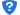## 2019年分类考试招生录取公示

我院2019年分类考试招生录取工作全部结束，考生可登录我院“2019年分类考试招生录取查询”系统（http://112.132.151.4:88/）输入个人信息查询。

通知书在5月底邮寄，邮寄地址和联系电话按考生当时报名填写的地址和电话。如果未能按时收到录取通知书，请电话联系招生就业处（电话：0558-5587000  3319700）。

新生开学报到时间为2019年9月5日—6日，报到时凭身份证和录取通知书现场办理入学手续。

温馨提示：近期有不法分子以学校名义要求考生缴纳学费或其他费用，请考生注意防范，不向任何索要费用的个人和单位汇款。新生缴费方式详见我院录取通知书。

【打印正文】

• 暂无资料
```

```
`	`

```

```
`	`

```

```
`	`

```

```
`	`

```

```
`	`

```

```
`	`

```

```
`	`

```

```
`	`

```

```
`	`

```

```
`	`

```

```
`	`

```

```
`	`

```

```
`	`

```

```
`	`

```

```
`	`

```

```
`	`

```

```
`	`

```

```
`	`

```

```
`	`

```

```
`	`

```

```
`	`

```

```
`	`

```

```
`	`

```

```
`	`

```

```
`	`

```

```
`	`

```

```
`	`

```

```
`	`

```

```
`	`

```

```
`	`

```

```
`	`

```

```
`	`

```

```
`	`

```

```
`	`

```

```
`	`

```

```
`	`

```

```
`	`

```

```
`	`

```

```
`	`

```

```
`	`

```

```
`	`

```

```
`	`

```

```
`	`

```

```
`	`

```

```
`	`

```

```
`	`

```

```
`	`

```

```
`	`

```

```
`	`

```

```
`	`

```

```
`	`

```

```
`	`

```

```
`	`

```

```
`	`

```

```
`	`

```

```
`	`

```

```
`	`

```

```
`	`

```

```
`	`

```

```
`	`

```

```
`	`

```

```
`	`

```

```
`	`

```

```
`	`

```

```
`	`

```

```
`	`

```

```
`	`

```

```
`	`

```

```
`	`

```

```
`	`

```

```
`	`

```

```
`	`

```

```
`	`

```

```
`	`

```

```
`	`

```

```
`	`

```

```
`	`

```

```
`	`

```

```
`	`

```

```
`	`

```

```
`	`

```

```
`	`

```

```
`	`

```

```
`	`

```

```
`	`

```

```
`	`

```

```
`	`

```

```
`	`

```

```
`	`

```

```
`	`

```

```
`	`

```

```
`	`

```

```
`	`

```

```
`	`

```

```
`	`

```

```
`	`

```

```
`	`

```

```
`	`

```

```
`	`

```

```
`	`

```

```
`	`

```

```
`	`

```

```
`	`

```

```
`	`

```

```
`	`

```

```
`	`

```

```
`	`

```

```
`	`

```

```
`	`

```

```
`	`

```

```
`	`

```

```
`	`

```

```
`	`

```

```
`	`

```

```
`	`

```

```
`	`

```

```
`	`

```

```
`	`

```

```
`	`

```

```
`	`

```

```
`	`

```

```
`	`

```

```
`	`

```

```
`	`

```

```
`	`

```

```
`	`

```

```
`	`

```

```
`	`

```

```
`	`

```

```
`	`

```

```
`	`

```

```
`	`

```

```
`	`

```

```
`	`

```

```
`	`

```

```
`	`

```

```
`	`

```

```
`	`

```

```
`	`

```

```
`	`

```

```
`	`

```

```
`	`

```

```
`	`

```

```
`	`

```

```
`	`

```

```
`	`

```

```
`	`

```

```
`	`

```

```
`	`

```

```
`	`

```

```
`	`

```

```
`	`

```

```
`	`

```

```
`	`

```

```
`	`

```

```
`	`

```

```
`	`

```

```
`	`

```

```
`	`

```

```
`	`

```

```
`	`

```

```
`	`

```

```
`	`

```

```
`	`

```

```
`	`

```

```
`	`

```

```
`	`

```

```
`	`

```

```
`	`

```

```
`	`

```

```
`	`

```

```
`	`

```

```
`	`

```

```
`	`

```

```
`	`

```

```
`	`

```

```
`	`

```

```
`	`

```

```
`	`

```

```
`	`

```

```
`	`

```

```
`	`

```

```
`	`

```

```
`	`

```

```
`	`

```

```
`	`

```

```
`	`

```

```
`	`

```

```
`	`

```

```
`	`

```

```
`	`

```

```
`	`

```

```
`	`

```

```
`	`

```

```
`	`

```

```
`	`

```

```
`	`

```

```
`	`

```

```
`	`

```

```
`	`

```

```
`	`

```

```
`	`

```

```
`	`

```

```
`	`

```

```
`	`

```

```
`	`

```

```
`	`

```

```
`	`

```

```
`	`

```

```
`	`

```

```
`	`

```

```
`	`

```

```
`	`

```

```
`	`

```

```
`	`

```

```
`	`

```

```
`	`

```

```
`	`

```

```
`	`

```

```
`	`

```

```
`	`

```

```
`	`

```

```
`	`

```

```
`	`

```

```
`	`

```

```
`	`

```

```
`	`

```

```
`	`

```

```
`	`

```

```
`	`

```

```
`	`

```

```
`	`

```

```
`	`

```

```
`	`

```

```
`	`

```

```
`	`